Disclosure: This post may contain affiliate links, meaning when you click the links and make a purchase, we receive a commission.

# How to find text in an Excel range and return cell reference (3 ways)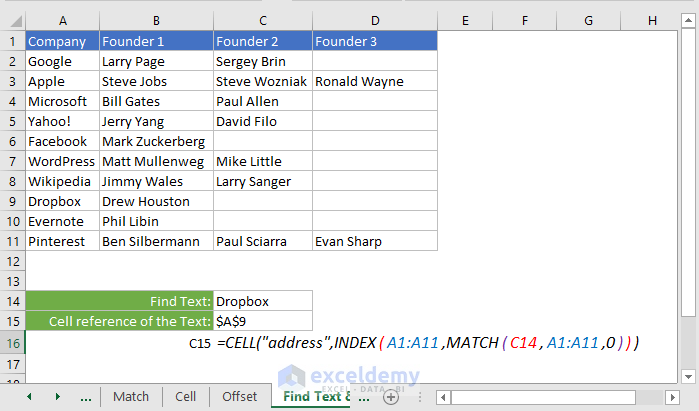In this tutorial, I shall write how to find text in an Excel range and return the reference of the cell that holds the text.

I will show several ways to do so. So that your requirement might match with any of the ways.

But before going into the main discussion, I want to discuss a little bit about the functions that I am going to use.

This is the Excel file that I have used to make this tutorial. Download and follow along with me.

## Pre-requisite Discussions

This part is optional for them who already are using heavily the following Excel functions:

• INDEX()
• MATCH()
• CELL()
• And OFFSET()

### # INDEX Function

INDEX function returns a value or reference of the cell at the intersection of a particular row and column, in a given range.

The syntax of INDEX Function:

INDEX(array, row_num, [column_num])

INDEX(reference, row_num, [column_num], [area_num])

Look at the image below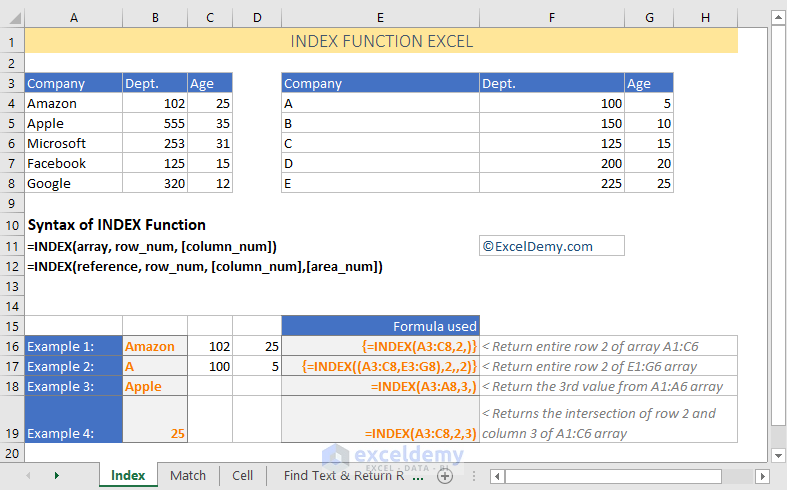Explanation of the formulas

Example 1

You might find Example 1 (and also Example 2) a little bit harder to understand. This is actually an Excel Array Formula.

To enter this formula, I have selected the cell range B16:D16 and then entered the formula in the cell B16.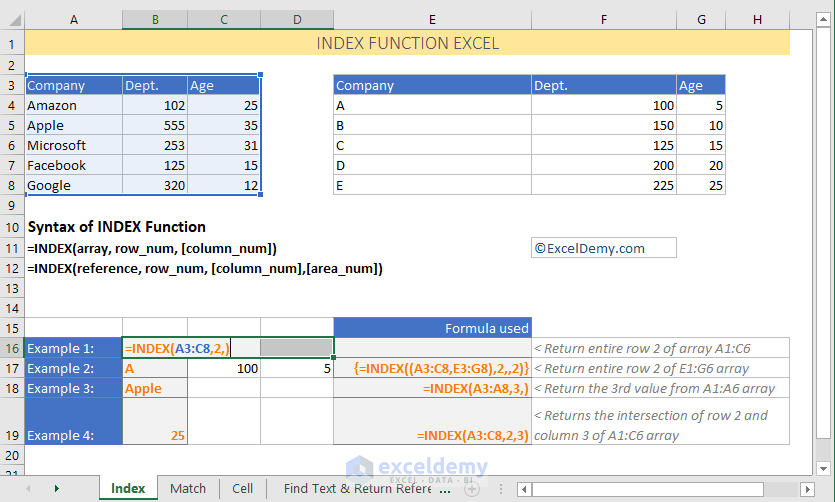Then I have pressed CTRL + SHIFT + ENTER to enter the array formula.

How this formula actually works?

{=INDEX(A3:C8,2,)}

Here the array part of the INDEX function is A3:C8. Its 2nd row is the A4:C4 row. As column number is blank, the INDEX function returns the entire 2nd row.

Example 2

{=INDEX((A3:C8,E3:G8),2,,2)}

• As the INDEX function reference, there are two ranges here: A3:C8 and E3:G8
• The row number is 2. No column number is specified. So, all the values of the 2nd row will be returned
• The range E3:G8 is used by the Index function as area number is 2

Example 3

=INDEX(A3:A8,3,)

It is a very simple INDEX formula. 3rd value of the array A3:A8 is returned by this formula.

Example 4

=INDEX(A3:C8,2,3)

This formula returns the intersection value 2nd row and 3rd column of the range A3:C8.

### # MATCH Function Excel

MATCH function returns the position of a value in an array of values.

The Syntax of MATCH function:

=MATCH(lookup_value, lookup_array, [match_type])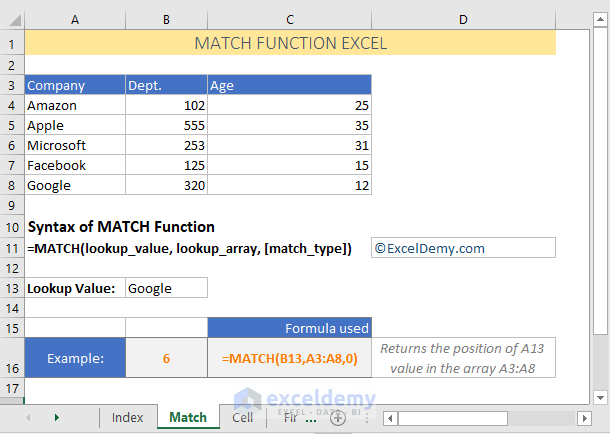How does this formula work?

=MATCH(B13,A3:A8,0)

• Value of cell B13 is Google. So, our lookup value is Google
• In the cell range A3:A8, the position of Google is 6th
• So, the formula returns 6

### # CELL Function Excel

CELL function returns information about the formatting, location, or contents of the first cell, according to the sheet’s reading order, in a reference.

The syntax of Excel CELL Function

=CELL(info_type, [reference])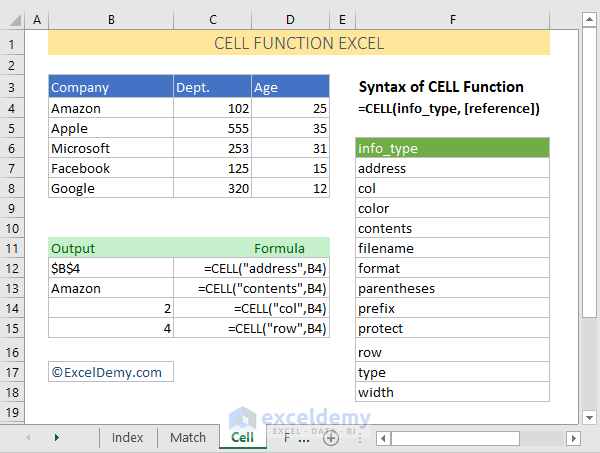Using CELL function, you can get a lot of details of a cell reference including the ABSOLUTE address. You can see it from the above image.

### # Excel OFFSET Function

Excel’s OFFSET function returns a reference to a range that is a given number of rows and columns from a given reference.

The syntax of OFFSET Function:

=OFFSET(reference, rows, cols, [height], [width])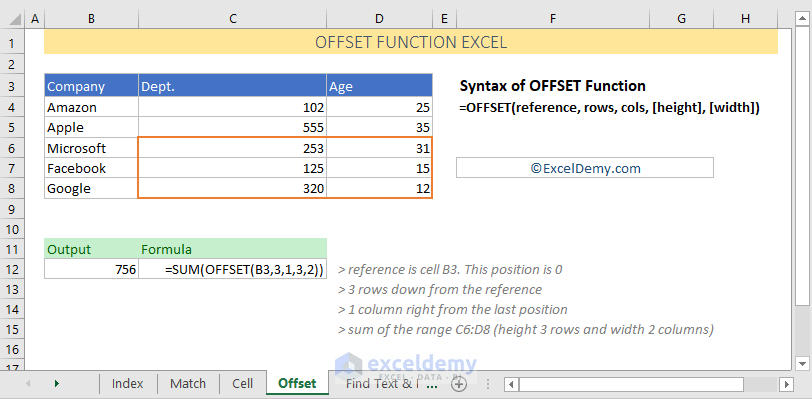How does this formula work?

=SUM(OFFSET(B3,3,1,3,2))

• Reference of the OFFSET function is the cell reference B3. So, cell B3’s position is 0.
• Then 3 rows down from the reference
• Then 1 column right from the last position
• Finally, the sum of the range C6:D8 (height 3 rows and width 2 columns). This returns value 756. The range C6:D8 is highlighted with an orange color border.

So, the pre-requisite discussion is over.

Now, let’s come to our main discussion.

## How to find text in an Excel range and return the cell reference

### Method 1: Text can be found in a single column range

In this method, we shall search the text in a single column and if found, the formula will return the reference.How does this formula work?

`=CELL("address",INDEX(A1:A11,MATCH(C14,A1:A11,0)))`

Let me explain the formula for the text “Dropbox”:

• This part of the formula, MATCH(C14,A1:A11,0), returns the value 9. Because the position of Dropbox in the array A1:A11 is 9th. So, overall formula becomes: `=CELL("address",INDEX(A1:A11,9))`
• Now INDEX(A1:A11,9) part refers to cell reference A9. So, the formula becomes:` =CELL("address",A9)`
• `=CELL("address",A9) `returns absolute reference of the cell A9.
• So, we get A9 as the output of the whole formula.

Note: INDEX(A1:A11,9) can return either the value or the cell reference. This is the beauty of the INDEX Function.

### Method 2: Text can be found in more than one column

In this method, we can search text from more than one column. But you have to select the column yourself.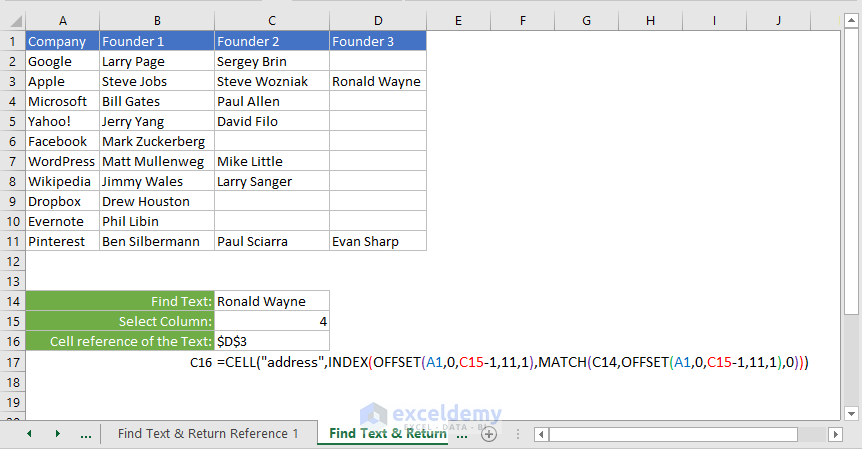I have used the following formula:

`=CELL("address",INDEX(OFFSET(A1,0,C15-1,11,1),MATCH(C14,OFFSET(A1,0,C15-1,11,1),0)))`

How does this formula work?

• This formula works like the above one. The only difference is: the column is selected dynamically using Excel’s OFFSET function. If you understand OFFSET function, then this part is simple to understand: OFFSET(A1,0,C15-1,11,1)

### Method 3: A single column might have more than one text

Sometimes a text value might repeat in a range more than once. We can return the row number of that text in the range.

You see from the following image that text “Apple” is repeating itself 3 times in the range A1:A11.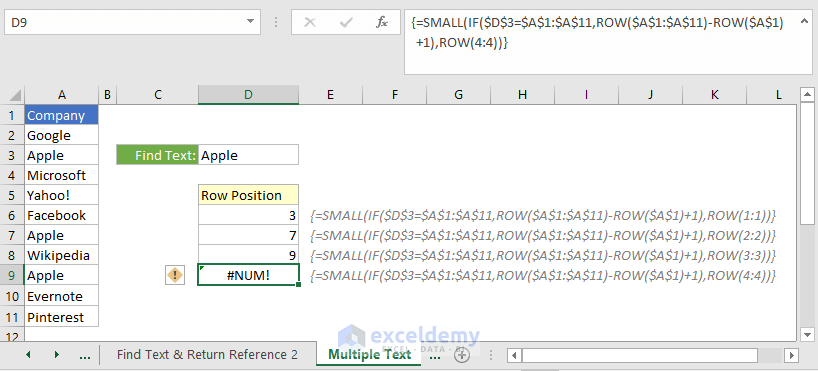Let me show you how I get these row numbers.

I have used this formula in the cell D6: {=SMALL(IF(\$D\$3=\$A\$1:\$A\$11,ROW(\$A\$1:\$A\$11)-ROW(\$A\$1)+1),ROW(1:1))}

Then I copied down this formula until the formula returns an error value.

It is clearly an Excel array formula.

But before, you have to know how the SMALL Function works in Excel.

The syntax of SMALL function:

SMALL(array,k)

For example, SMALL({80;35;55;900},2) will return the 2nd smallest value in the array {80;35;55;900}. The output will be: 55

So, how does the formula work?

Cell D6 = {=SMALL(IF(\$D\$3=\$A\$1:\$A\$11,ROW(\$A\$1:\$A\$11)-ROW(\$A\$1)+1),ROW(1:1))}

To understand this array formula clearly, you can read my guide: Excel Array Formula Basic 2 – Breakdown of Array Formula

• This part of the formula, IF(\$D\$3=\$A\$1:\$A\$11,ROW(\$A\$1:\$A\$11)-ROW(\$A\$1)+1), actually returns the array for the small function.
• Logical test part is: \$D\$3=\$A\$1:\$A\$11. This part tests (one by one) whether the values of the range \$A\$1:\$A\$11 is equal to \$D\$3 or not. If equal, a TRUE value is set in the array and if not equal, a False value is set in the array: {FALSE;FALSE;TRUE;FALSE;FALSE;FALSE;TRUE;FALSE;TRUE;FALSE;FALSE}
• And value_if_true part is: ROW(\$A\$1:\$A\$11)-ROW(\$A\$1)+1). This whole part returns something like this: {1;2;3;4;5;6;7;8;9;10;11} – {1} + 1 = {0;1;2;3;4;5;6;7;8;9;10} + 1 = {1;2;3;4;5;6;7;8;9;10;11}
• ROW(1:1) is actually the k of the SMALL function. And it returns 1.
• So, the formula in the cell D6 becomes like this: SMALL(IF({FALSE;FALSE;TRUE;FALSE;FALSE;FALSE;TRUE;FALSE;TRUE;FALSE;FALSE},{1;2;3;4;5;6;7;8;9;10;11}),1)
• Now the IF function returns this array: {FALSE;FALSE;3;FALSE;FALSE;FALSE;7;FALSE;9;FALSE;FALSE}
• The formula becomes: SMALL({FALSE;FALSE;3;FALSE;FALSE;FALSE;7;FALSE;9;FALSE;FALSE},1)
• Finally, the formula returns 3

I hope you get how this complex formula works.

## Conclusion

So, that’s all about finding text in an Excel range and then returning the cell address or row references (if the text repeat more than once in the range).

Thanks for reading our blog. If you have comments or feedback on this article, let us know in the comment box. Your feedback inspires us to create more documents for Excel.